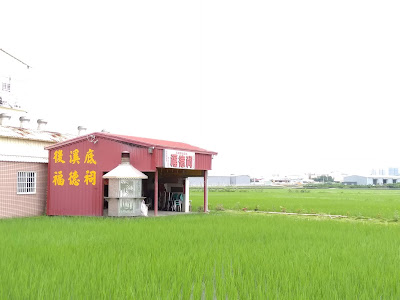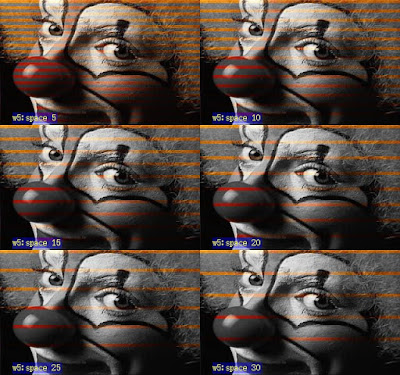## 文章

### 用Javascript製作視野測定的程式### 到台中霧峰看看為猛禽搭的架### 用循環紙圈記憶分析血液循環途徑### 用漱口水取得的大片口腔皮膜組織### 用Imagej製作灰階影像加彩色線條的視覺效果run("Select None"); rename("color"); run("Duplicate...", "title=grey");
selectWindow("grey"); run("8-bit"); run("RGB Color"); run("Set Measurements...", "area bounding redirect=None decimal=3"); roiManager("Select", 0); run("Rotate...", "angle="+ angle +""); roiManager("Add"); run("To Bounding Box"); run("Measure");
BX = getResult("BX", nResults-1); BY = getResult("BY", nResults-1); Width = getResult("Width", nResults-1); Height = getResult("Heigh…

### 用Imagej做顯微追焦=====用點標記的======
origin_x= getWidth/2;
origin_y= getHeight/2;
for (i = 0; i <  nResults ; i++) {
slice_x = getResult("X", i);
slice_y = getResult("Y", i);

translate_x= origin_x-slice_x;
translate_y= origin_y-slice_y;

setSlice(getResult("Slice", i));
run("Translate...", "x="+translate_x+" y="+translate_y+" interpolation=None slice");
}

====用線標記的=========
origin_x= getWidth/2;
origin_y= getHeight/2;
for (i = 0; i <  nResults ; i++) {
BX =  getResult("BX", i);
BY =  getResult("BY", i);
W =  getResul…